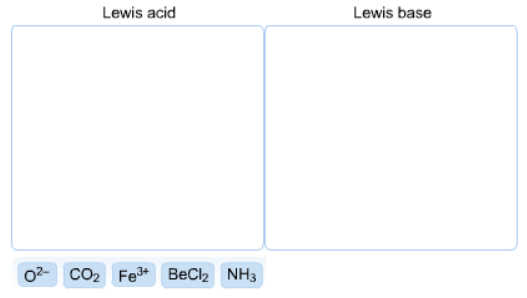Problem: Classify each of the following as a Lewis acid or a Lewis base.

86% (71 ratings)
Problem Details

Classify each of the following as a Lewis acid or a Lewis base.What scientific concept do you need to know in order to solve this problem?

Our tutors have indicated that to solve this problem you will need to apply the Lewis Acid and Base concept. You can view video lessons to learn Lewis Acid and Base Or if you need more Lewis Acid and Base practice, you can also practice Lewis Acid and Base practice problems .

What is the difficulty of this problem?

Our tutors rated the difficulty of Classify each of the following as a Lewis acid or a Lewis ba... as medium difficulty.

How long does this problem take to solve?

Our expert Chemistry tutor, Sabrina took 5 minutes to solve this problem. You can follow their steps in the video explanation above.

What professor is this problem relevant for?

Based on our data, we think this problem is relevant for Professor Henricks' class at KSU.